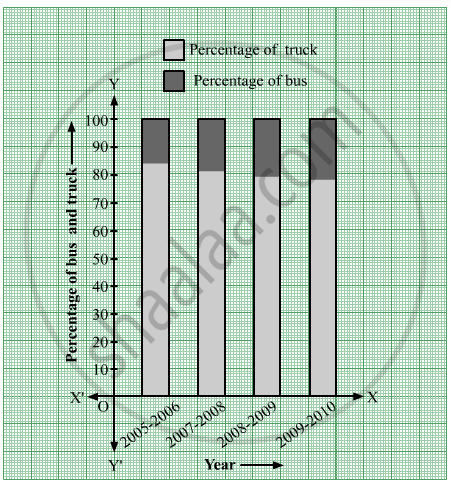Share

# The Following Table Shows the Number of Buses and Trucks in Nearest Lakh Units. Draw Percentage Bar-diagram. (Approximate the Percentages to the Nearest Integer) - Algebra

ConceptBar Diagrams

#### Question

The following table shows the number of Buses and Trucks in nearest lakh units. Draw percentage bar-diagram. (Approximate the percentages to the nearest integer)

 Year No. of Trucks No. of Buses 2005-2006 47 9 2007-2008 56 13 2008-2009 60 16 2009-2010 63 18

#### Solution

The following table shows the number of Buses and Trucks in nearest lakh units.

 Year No  of trucks Percentage of trucks No of buses Percentage of buses 2005-2006 47 = 47/ ( 47 + 9 ) x 100 = 4700 / 56 = 83. 92 ≈ 84 9 = 9 / ( 47 + 9 ) x 100 =  900 / 56  = 16.07 ≈ 16 2007-2008 56 =  56/ ( 56 + 13) x 100 =  5600 / 69 = 81.15 ≈ 81 13 = 13 / ( 56 + 13) x 100 = 1300/69 = 18.84 ≈ 19 2008-2009 60 =  60 / ( 60 + 16 )  x100 = 6000 / 76 = 78.94 ≈ 79 16 =  16 / ( 60+ 16 ) x 100 = 1600 / 76 = 21.05 ≈ 21 2009-2010 63 = 63 / ( 63 + 18 ) x 100 = 6300/ 81= 77.77 ≈ 78 18 =  18 / ( 63 + 18 ) x 100 = 1800 / 81  = 22.22 ≈ 22

The percentage bar-diagram of the above table is as follows:Is there an error in this question or solution?

#### APPEARS IN

Balbharati Solution for Balbharati Class 9 Mathematics 1 Algebra (2019 to Current)
Chapter 7: Statistics
Practice set 7.1 | Q: 1 | Page no. 111
Solution The Following Table Shows the Number of Buses and Trucks in Nearest Lakh Units. Draw Percentage Bar-diagram. (Approximate the Percentages to the Nearest Integer) Concept: Bar Diagrams.
S